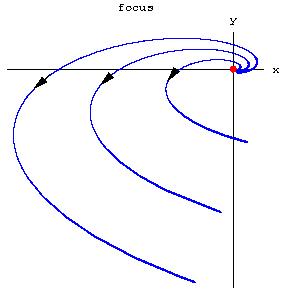# Focus

A type of arrangement of the trajectories of an autonomous system of first-order ordinary differential equations

$$\tag{* } \dot{x} = f ( x),\ \ x = ( x _ {1} , x _ {2} ),\ \ f: G \subset \mathbf R ^ {2} \rightarrow \mathbf R ^ {2} ,$$

$f \in C ( G)$, where $G$ is the domain of uniqueness, in a neighbourhood of a singular point $x _ {0}$( cf. Equilibrium position). This type is characterized as follows. There is a neighbourhood $U$ of $x _ {0}$ such that for all trajectories of the system starting in $U \setminus \{ x _ {0} \}$, the negative semi-trajectories are escaping (in the course of time they leave any compact set $V \subset U$) and the positive semi-trajectories, without leaving $U$, tend to $x _ {0}$, winding round it like a logarithmic spiral, or conversely. The point $x _ {0}$ itself is also called a focus. The nature of the approach of the trajectories of the system to $x _ {0}$ can be described more precisely if one introduces polar coordinates $r, \phi$ on the $( x _ {1} , x _ {2} )$- plane with pole at $x _ {0}$. Then for any semi-trajectory $r = r ( t)$, $\phi = \phi ( t)$, $t \geq 0$( $t \leq 0$), that tends to $x _ {0}$, the polar angle of the variable point $\phi ( t) \rightarrow + \infty$( a left focus) or $- \infty$( a right focus) as $t \rightarrow \infty$.

A focus is either asymptotically Lyapunov stable or completely unstable (asymptotically stable as $t \rightarrow - \infty$). Its Poincaré index is 1. The figure depicts a right unstable focus at $x _ {0} = ( 0, 0)$.Figure: f040700a

For a system (*) of class $C ^ {1}$( $f \in C ^ {1} ( G)$) a singular point $x _ {0}$ is a focus in case the matrix $A = f ^ { \prime } ( x _ {0} )$ has complex conjugate eigen values with non-zero real part, but it may also be a focus in case this matrix has purely imaginary or multiple real eigen values (see also Centre; Centre and focus problem).

For references see Singular point of a differential equation.